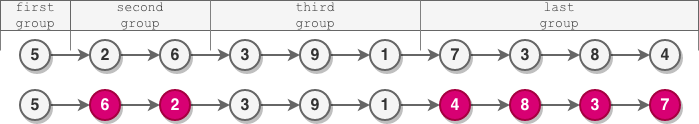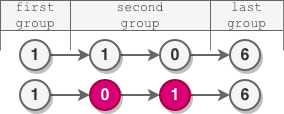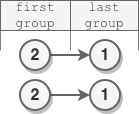Formatted question description: https://leetcode.ca/all/2074.html

# 2074. Reverse Nodes in Even Length Groups (Medium)

You are given the head of a linked list.

The nodes in the linked list are sequentially assigned to non-empty groups whose lengths form the sequence of the natural numbers (1, 2, 3, 4, ...). The length of a group is the number of nodes assigned to it. In other words,

• The 1st node is assigned to the first group.
• The 2nd and the 3rd nodes are assigned to the second group.
• The 4th, 5th, and 6th nodes are assigned to the third group, and so on.

Note that the length of the last group may be less than or equal to 1 + the length of the second to last group.

Reverse the nodes in each group with an even length, and return the head of the modified linked list.

Example 1:Input: head = [5,2,6,3,9,1,7,3,8,4]
Output: [5,6,2,3,9,1,4,8,3,7]
Explanation:
- The length of the first group is 1, which is odd, hence no reversal occurrs.
- The length of the second group is 2, which is even, hence the nodes are reversed.
- The length of the third group is 3, which is odd, hence no reversal occurrs.
- The length of the last group is 4, which is even, hence the nodes are reversed.


Example 2:Input: head = [1,1,0,6]
Output: [1,0,1,6]
Explanation:
- The length of the first group is 1. No reversal occurrs.
- The length of the second group is 2. The nodes are reversed.
- The length of the last group is 1. No reversal occurrs.


Example 3:Input: head = [2,1]
Output: [2,1]
Explanation:
- The length of the first group is 1. No reversal occurrs.
- The length of the last group is 1. No reversal occurrs.


Example 4:

Input: head = 
Output: 
Explanation: There is only one group whose length is 1. No reversal occurrs.


Constraints:

• The number of nodes in the list is in the range [1, 105].
• 0 <= Node.val <= 105

Similar Questions:

## Solution 1. Two Pass

// OJ: https://leetcode.com/problems/reverse-nodes-in-even-length-groups/
// Time: O(N)
// Space: O(1)
class Solution {
int getLength(ListNode *head) {
int len = 0;
return len;
}
public:
ListNode* reverseEvenLengthGroups(ListNode* head) {
ListNode dummy, *tail = &dummy;
for (int i = 0, total = getLength(head); head; ++i) {
int len = min(total, i + 1); // the length of the current section
ListNode *newTail = NULL; // The new tail if we need to reverse the current section
for (int j = 0; j < len && head; ++j) {
auto p = head;
if (len % 2) { // direct append
tail->next = p;
tail = p;
} else { // append in reverse order
if (newTail == NULL) newTail = p;
p->next = tail->next;
tail->next = p;
}
}
if (newTail) tail = newTail;
tail->next = NULL;
total -= len;
}
return dummy.next;
}
};


## Discuss

https://leetcode.com/problems/reverse-nodes-in-even-length-groups/discuss/1576917/C%2B%2B-Two-Pass